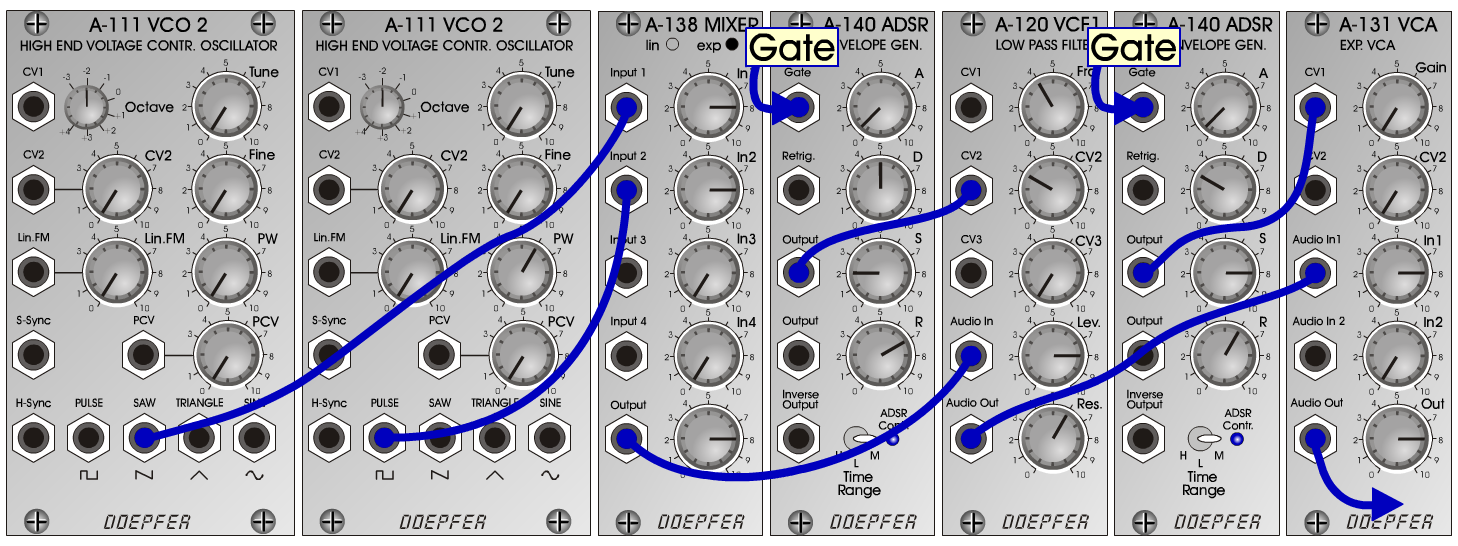Plasma-BassInstrumentsD o e p f e r A - 100 Connections: Settings Plasma-Bass 1: A-111/1 (Saw)  <=>  A-138exp (Input 1) A-111/2 (Pulse)  <=>  A-138exp (Input 2) A-138exp (Output)  <=>  A-120 (Audio In) A-140/1 (Output)  <=>  A-120 (CV2) A-120 (Audio Out)  <=>  A-131 (Audio In1) A-140/2 (Output)  <=>  A-131 (CV1) A-131 (Audio Out)  <=> Amplifier A-111/2 (PW = 6) A-140/1 (A = 0.5, D = 5, S = 2, R = 7, Range = M) A-120 (Frq = 4, CV2  = 3, Lev = 8, Res = 6) A-140/2 (A = 0.5, D = 3, S = 8, R = 6, Range = M) A-131 (Gain = 0, In 1 = 8,  Audio Out = 8) Settings Plasma-Bass 2: A-111/2 (PW = 6) A-140/1 (A = 0.5, D = 5, S = 2, R = 7, Range = M) A-120 (Frq = 2, CV2  = 4, Lev = 8, Res = 6) A-140/2 (A = 0.5, D = 6, S = 0, R = 4, Range = M) A-131 (Gain = 0, In 1 = 8,  Audio Out = 8) Settings Plasma-Bass 3: A-111/2 (PW = 6) A-140/1 (A = 0.5, D = 5, S = 2, R = 7, Range = M) A-120 (Frq = 4, CV2  = 5, Lev = 8, Res = 6) A-140/2 (A = 0.5, D = 6, S = 0, R = 4, Range = M) A-131 (Gain = 0, In 1 = 8,  Audio Out = 8) Notes: Tune A-111/2 10 cents higher then A-111/1. Josef MuellerSound samples Plasma-Bass-Piece 1 Plasma-Bass-Piece 1 (reverb) Plasma-Bass-Piece 2 Plasma-Bass-Piece 2 (chorus) Plasma-Bass-Piece 3 Plasma-Bass-Piece 3 (reverb) Plasma-Bass 1 (98 Hz) Plasma-Bass 1 (131 Hz) Plasma-Bass 1 (196 Hz) Plasma-Bass 2 (98 Hz) Plasma-Bass 2 (131 Hz) Plasma-Bass 2 (196 Hz) Plasma-Bass 3 (98 Hz) Plasma-Bass 3 (131 Hz) Plasma-Bass 3 (196 Hz)